# WA Continuous Wavelet Transform VI

LabVIEW 2014 Advanced Signal Processing Toolkit Help

Edition Date: June 2014

Part Number: 372656C-01

»View Product InfoDownload Help (Windows Only)

Owning Palette: Continuous Wavelet VIs

Computes the continuous wavelet transform (CWT) of the 1D input signal with real-valued wavelets. Wire data to the signal input to determine the polymorphic instance to use or manually select the instance.

Use the pull-down menu to select an instance of this VI.

 Select an instance WA Continuous Wavelet Transform (Waveform)WA Continuous Wavelet Transform (Array)

## WA Continuous Wavelet Transform (Waveform)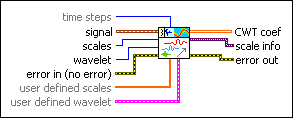time steps specifies the number of samples to translate, or shift, the wavelet in the continuous wavelet transform (CWT). The default is –1, which specifies that this VI adjusts time steps automatically so that no more than 512 coefficients exist at each scale. The number of rows in the output wavelet coefficients equals the signal length divided by time steps.

National Instruments recommends that you set time steps such that the number of rows in the wavelet coefficients does not exceed 512. If you specify a small value for time steps, this VI might return a large number of wavelet coefficients, which requires a long computation time and more memory.

If you need a small time step to observe more details and the signal length is large, divide the signal into smaller segments and compute the wavelet coefficients for each segment. If the signal is oversampled, you can downsample the signal. time steps must be greater than 0, or this VI sets time steps to the default value –1 automatically.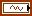signal specifies the input signal.scales specifies the number of scales of the dilated wavelet.wavelet specifies the wavelet type to use to compute the continuous wavelet coefficients. The default is db02. The options include the following types: Mexican Hat, Meyer, Morlet, orthogonal (Haar, Daubechies (dbxx), Coiflets (coifx), Symmlets (symx)) and biorthogonal (Biorthogonal (biorx_x), including FBI (bior4_4 (FBI))), where x indicates the order of the wavelet. wavelet must be the same type as the wavelet type you selected in the WA Arbitrary Path Decomposition VI to compute path coef and residual info. If you used the analysis filters input of the WA Arbitrary Path Decomposition VI to specify wavelet, ignore this input and use the synthesis filters input of this VI to specify the synthesis filters of the wavelet. Use the user defined wavelet input to specify a customized wavelet.error in describes error conditions that occur before this node runs. This input provides standard error in functionality.user defined scales specifies the scales to use to compute CWT coef. The scale must be positive and no greater than the length of signal. If you specify a value for user defined scales, this VI ignores the settings in the scales input.user defined wavelet specifies the mother wavelet function to use to compute the continuous wavelet transform (CWT). If you specify a value for user defined wavelet, this VI ignores the settings in the wavelet input. You can use the Wavelet Design Express VI to design the wavelet.t0 specifies the initial time of the mother wavelet function.dt specifies the time interval of the mother wavelet function in samples.mother wavelet specifies the mother wavelet function.CWT coef returns the results of the continuous wavelet transform (CWT). The element in the ith column and the jth row is the result of the CWT, where scale (a)=i+1, and shift ()=j×time steps. When you use user defined scales to define the scales of the CWT, the element in the ith column and the jth row is the result of the CWT, where scale (a) equals the ith element of user defined scales and shift () equals j×time steps. The squared magnitude of CWT coef is the scalogram, which jointly represents a signal in terms of time and scale. Large scales correspond to low frequencies, and small scales correspond to high frequencies. You also can consider the scalogram as the time-frequency representation of the signal. Use the WA Scalogram Indicator to display the scalogram on an intensity graph.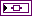scale info returns the time information and the scale (frequency) information, which this VI uses in the scalogram plot.error out contains error information. This output provides standard error out functionality.

## WA Continuous Wavelet Transform (Array)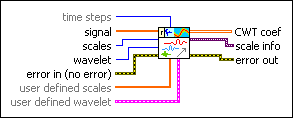time steps specifies the number of samples to translate, or shift, the wavelet in the continuous wavelet transform (CWT). The default is –1, which specifies that this VI adjusts time steps automatically so that no more than 512 coefficients exist at each scale. The number of rows in the output wavelet coefficients equals the signal length divided by time steps.

National Instruments recommends that you set time steps such that the number of rows in the wavelet coefficients does not exceed 512. If you specify a small value for time steps, this VI might return a large number of wavelet coefficients, which requires a long computation time and more memory.

If you need a small time step to observe more details and the signal length is large, divide the signal into smaller segments and compute the wavelet coefficients for each segment. If the signal is oversampled, you can downsample the signal. time steps must be greater than 0, or this VI sets time steps to the default value –1 automatically.signal specifies the input signal.scales specifies the number of scales of the dilated wavelet.wavelet specifies the wavelet type to use to compute the continuous wavelet coefficients. The default is db02. The options include the following types: Mexican Hat, Meyer, Morlet, orthogonal (Haar, Daubechies (dbxx), Coiflets (coifx), Symmlets (symx)) and biorthogonal (Biorthogonal (biorx_x), including FBI (bior4_4 (FBI))), where x indicates the order of the wavelet. wavelet must be the same type as the wavelet type you selected in the WA Arbitrary Path Decomposition VI to compute path coef and residual info. If you used the analysis filters input of the WA Arbitrary Path Decomposition VI to specify wavelet, ignore this input and use the synthesis filters input of this VI to specify the synthesis filters of the wavelet. Use the user defined wavelet input to specify a customized wavelet.error in describes error conditions that occur before this node runs. This input provides standard error in functionality.user defined scales specifies the scales to use to compute CWT coef. The scale must be positive and no greater than the length of signal. If you specify a value for user defined scales, this VI ignores the settings in the scales input.user defined wavelet specifies the mother wavelet function to use to compute the continuous wavelet transform (CWT). If you specify a value for user defined wavelet, this VI ignores the settings in the wavelet input. You can use the Wavelet Design Express VI to design the wavelet.t0 specifies the initial time of the mother wavelet function.dt specifies the time interval of the mother wavelet function in samples.mother wavelet specifies the mother wavelet function.CWT coef returns the results of the continuous wavelet transform (CWT). The element in the ith column and the jth row is the result of the CWT, where scale (a)=i+1, and shift ()=j×time steps. When you use user defined scales to define the scales of the CWT, the element in the ith column and the jth row is the result of the CWT, where scale (a) equals the ith element of user defined scales and shift () equals j×time steps. The squared magnitude of CWT coef is the scalogram, which jointly represents a signal in terms of time and scale. Large scales correspond to low frequencies, and small scales correspond to high frequencies. You also can consider the scalogram as the time-frequency representation of the signal. Use the WA Scalogram Indicator to display the scalogram on an intensity graph.scale info returns the time information and the scale (frequency) information, which this VI uses in the scalogram plot.error out contains error information. This output provides standard error out functionality.

## WA Continuous Wavelet Transform Details

The following equation defines the CWT: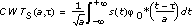where s(t) is the signal,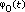is the mother wavelet function, and a andare the scale and shift of the wavelet, respectively. a can be any positive real value. Use user defined scales to specify the scales. If you do not specify values for user defined scales, this VI selects the scale, a, as 1, 2, 3, …, scales. This VI selects the shift,, as 0, dt, 2dt, 3dt, …, Ndt, where dt is time steps, and N is approximately equal to the signal length divided by time steps. Refer to A Wavelet Tour of Signal Processing for more information about the CWT.

## Example

Refer to the Breakdown Point Detection VI in the labview\examples\Wavelet Analysis\WAApplications directory for an example of using the WA Continuous Wavelet Transform VI.